# Balancing Chemical Equations Balancing Chemical Equations To describe

• Slides: 16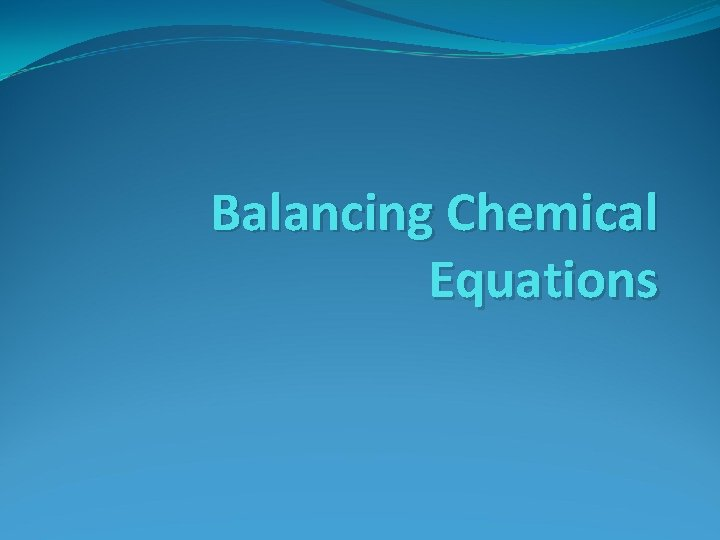Balancing Chemical EquationsBalancing Chemical Equations To describe a reaction accurately, a chemical equation must show the same number of each type of atom on both sides of the equation. Chemists say an equation is balanced when it accurately represents Conservation of MassHow to Balance a Chemical Equation 1. 2. 3. 4. Write the equation Count the atoms Use coefficients to balance atoms Look back and check1. Write the Equation Write the correct formulas for both the reactants and products. H 2 + O 2 H 2 O Reactants Products Place the reactants, H 2 and O 2, on the left side of the arrow, separated by a plus sign. Next, write the product, H 2 O, on the right side of the arrow.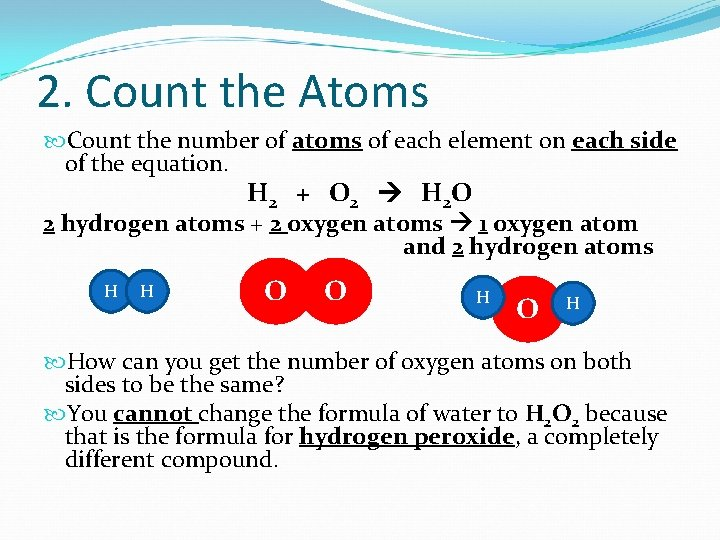2. Count the Atoms Count the number of atoms of each element on each side of the equation. H 2 + O 2 H 2 O 2 hydrogen atoms + 2 oxygen atoms 1 oxygen atom and 2 hydrogen atoms H H O O H How can you get the number of oxygen atoms on both sides to be the same? You cannot change the formula of water to H 2 O 2 because that is the formula for hydrogen peroxide, a completely different compound.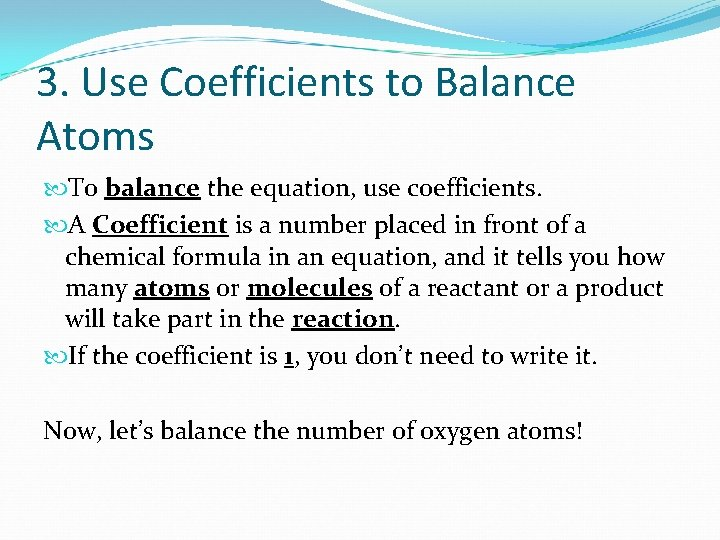3. Use Coefficients to Balance Atoms To balance the equation, use coefficients. A Coefficient is a number placed in front of a chemical formula in an equation, and it tells you how many atoms or molecules of a reactant or a product will take part in the reaction. If the coefficient is 1, you don’t need to write it. Now, let’s balance the number of oxygen atoms!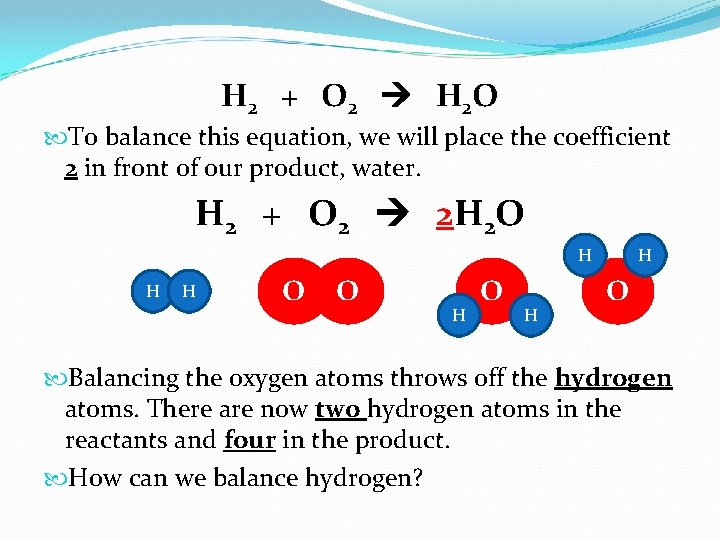H 2 + O 2 H 2 O To balance this equation, we will place the coefficient 2 in front of our product, water. H 2 + O 2 2 H 2 O H H H O O H H O Balancing the oxygen atoms throws off the hydrogen atoms. There are now two hydrogen atoms in the reactants and four in the product. How can we balance hydrogen?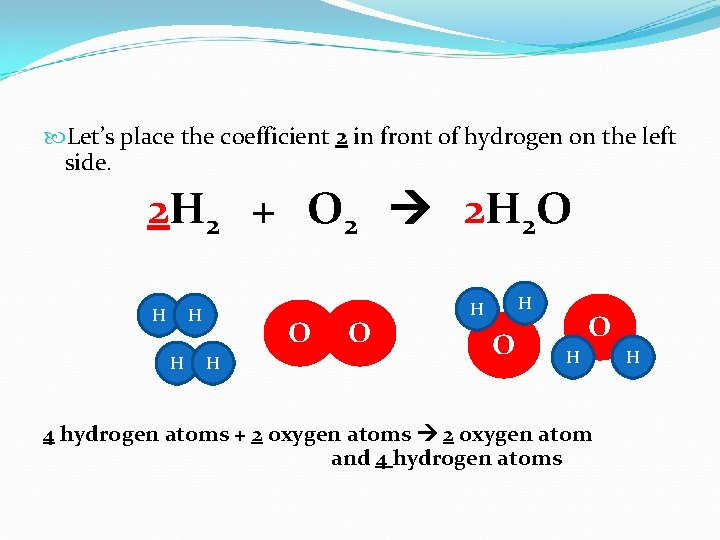Let’s place the coefficient 2 in front of hydrogen on the left side. 2 H 2 + O 2 2 H 2 O H H H O O H 4 hydrogen atoms + 2 oxygen atoms 2 oxygen atom and 4 hydrogen atoms H4. Look Back and Check We can make sure the equation is balanced by counting the atoms of each element on each side. 2 H 2 + O 2 2 H 2 O This equation tells us that two molecules of hydrogen react with one molecule of oxygen to yield two molecules of water.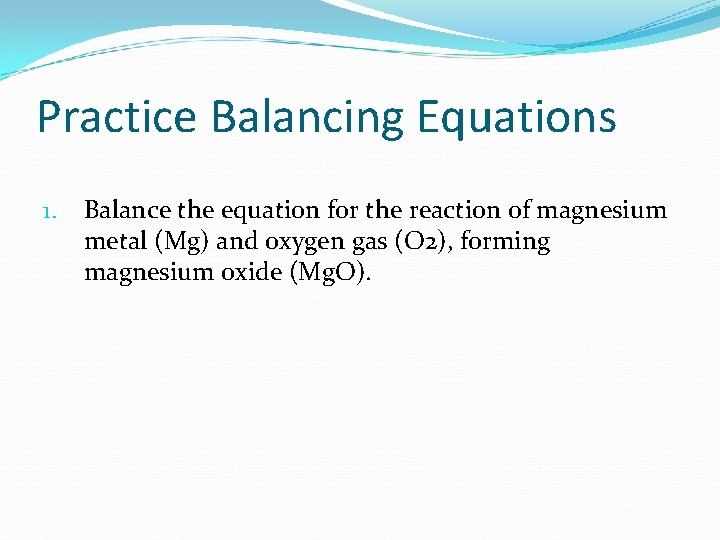Practice Balancing Equations 1. Balance the equation for the reaction of magnesium metal (Mg) and oxygen gas (O 2), forming magnesium oxide (Mg. O).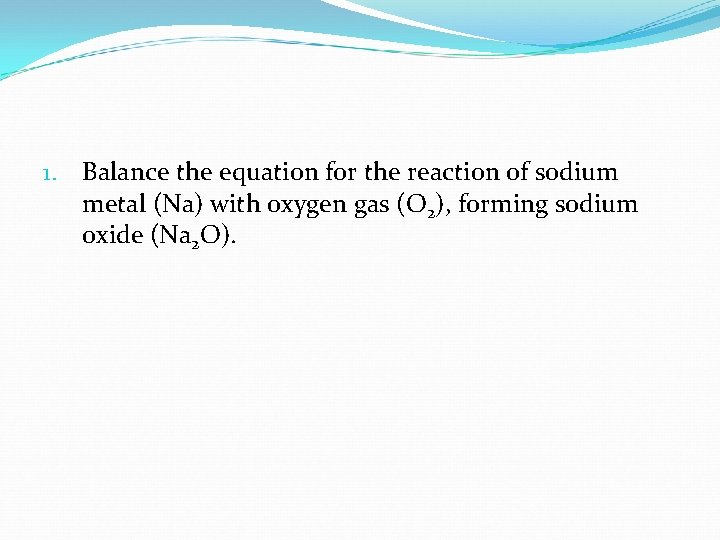1. Balance the equation for the reaction of sodium metal (Na) with oxygen gas (O 2), forming sodium oxide (Na 2 O).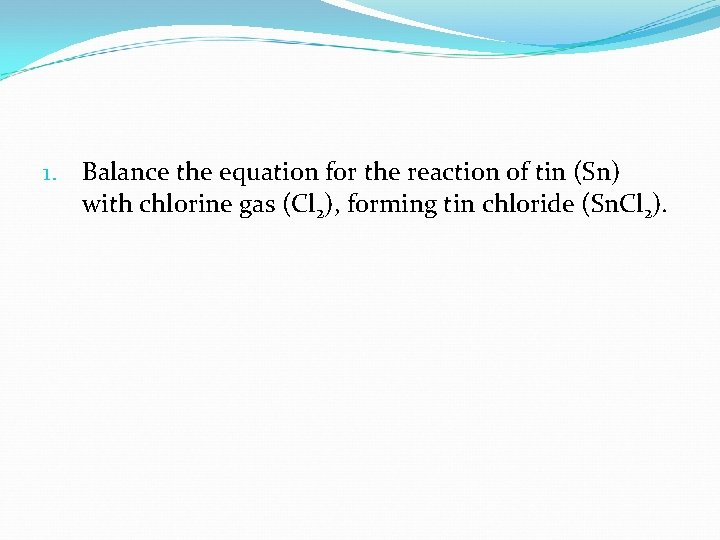1. Balance the equation for the reaction of tin (Sn) with chlorine gas (Cl 2), forming tin chloride (Sn. Cl 2).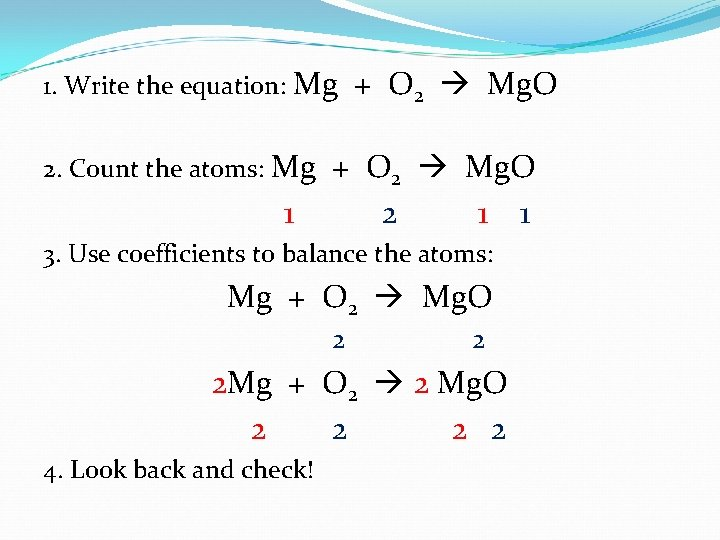1. Write the equation: Mg 2. Count the atoms: Mg 1 + O 2 Mg. O 2 1 1 3. Use coefficients to balance the atoms: Mg + O 2 Mg. O 2 2 2 Mg + O 2 2 Mg. O 2 2 4. Look back and check!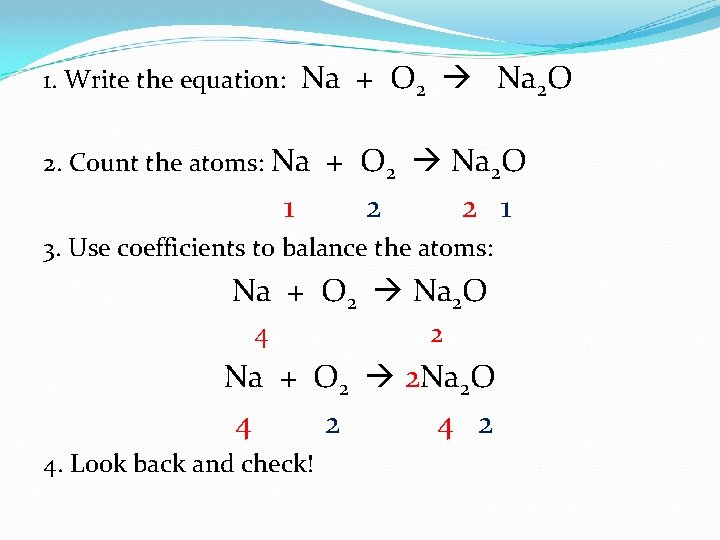1. Write the equation: Na + O 2 Na 2 O 2. Count the atoms: Na 1 + O 2 Na 2 O 2 2 1 3. Use coefficients to balance the atoms: Na + O 2 Na 2 O 4 2 Na + O 2 2 Na 2 O 4 2 4. Look back and check!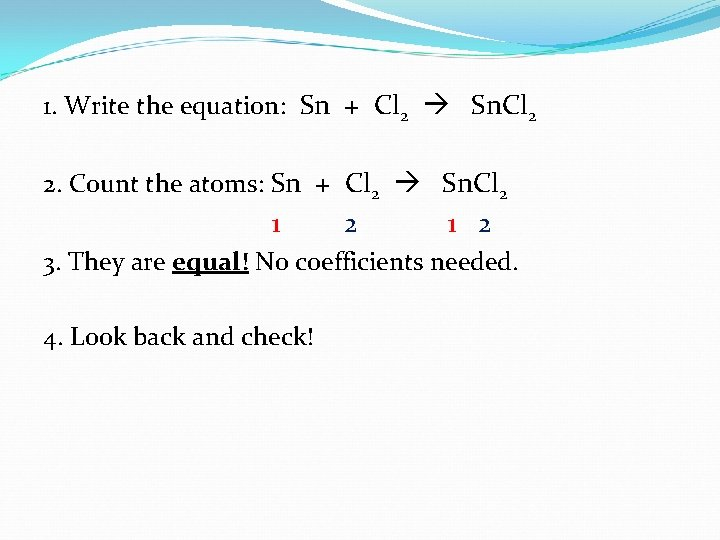1. Write the equation: Sn + Cl 2 Sn. Cl 2 2. Count the atoms: Sn + Cl 2 Sn. Cl 2 1 2 3. They are equal! No coefficients needed. 4. Look back and check!